# Numerical study of entropy generation and natural convection heat transfer in trapezoidal enclosure with a thin baffle attached to inner wall using liquid nanofluid

Numerical study of entropy generation and natural convection heat transfer in trapezoidal enclosure with a thin baffle attached to inner wall using liquid nanofluid

Air conditioning and Refrigeration Techniques Engineering Department, Al-Mustaqbal University College, Babylon, 51001, Iraq

Department of mechanical engineering, Babylon University, Babylon, 51001, Iraq

Corresponding Author Email:
Page:
7-28
|
DOI:
https://doi.org/10.3166/ACSM.41.7-28
| |
Accepted:
| | Citation

OPEN ACCESS

Abstract:

The present work, the natural convection and the entropy generation of trapezoidal enclosure with an embedded baffle using Cu nanofluids are numerically studied.  The governing equations of fluid heat transfer and fluid mechanics like continuty, energy and momentum of the fluid has been solved numerically using the finite element method. The impact of many dimesnionless parameters such as Rayleigh number, three different cases of baffle height (CASE-1, CASE-2, and CASE-3) on streamlines, isotherms, entropy generation, local and the average Nusselt number is presented for Cu nanofluid. The results indicate that as the Rayleigh number goes up, fluid flow strength will increase and heat transfer will enhance. Also, at high Rayleigh number, the entropy generation due to fluid friction will be greater than that due to heat transfer. Finally, it is obtained that CASE-1 gives better heat transfer characterize in a comparison with other cases of baffle height

Keywords:

natural convection, baffle, nanofluid, enclosure

1. Introduction

The baffle involve on the natural convection filled with base fluid was studied by. Bilgen (2005) used square enclosure filled with air to examin the natural convection for differentially heated on vertical walls with an attached solid thin fin to the hot wall. The obtained results showed that the mean Nusselt number is fependent upon the fin length and its position. The impact of the inclination angle of this heated fin and its length on streamlines and isotherms were presented in (Ben-Nakhi & Chamkha, 2006) and they reported that Rayleigh number, inclination fin and its length have a considerable impact on the average Nusselt number. The existence of a divider located within square cavity full of pure fluid with volumetric internal heat generation is investigated numerically by (Oztop & Bilgen, 2006). The phenomenon of natural convective in a tilted enclsoure with a fluffy heat generated baffle located in the middle of rectangular packaging is studied numerically by (Altaç & Kurtul, 2007). Chahrazed and Samir (2012) numerically examined the natural convectove flow within sqaure cavity under transient conditions full of air with two baffles attached to the worm wall. A correlation had been reported to predict a Nusselt number as a function of fin length and it is found that the effectiveness of the fin was improved by increasing its length.

The baffle impact on the natural convection filled with nanofluid was investigated by. Mahmoudi et al., (2010) demonstrated the natural convection within square enclosure filled with copper-water nanofluid with horizontal thin fin treated as a heat source. The effect of adopting parameters like Rayleigh number, nanofluid concentrations, length and positions of the baffle on streamlines and the Nusselt number is investigated. Effects of various types of nanofluid on a square enclosure containing a thin fin is examined by (Mahmoodi, 2011). The results indicated that Ag-water nanofluid gives better heat transfer characteristics more than the other types. Also, it is obtained that at low values Rayleigh numbers the horizontal heated thin fin has a higher Nusselt number in a comparison with that of vertical positioned. However, at altitude values of Rayleigh numbers, the fin position does not impact the rate of heat transfer. The conjugate (conduction-conductive) heat transfer problem in a divided square enclosure full of two different types of nanofluid on its two sides is investigated using finite element techniques numerically. The impact of many dimensionless parameters like Grashof number, inclination angle, the position of the fin and nanofluid concentration on heat transfer and streamlines is presented in (Selimefendigil & Öztop, 2016).

A number of studies regarding trapezoidal cavity full of pure fluid (Iyican et al., 1980; Karyakin, 1989; Lee, 1991; Perić, 1993; Kuyper & Hoogendoorn, 1995; Karki, 1987). Also, trapezoidal filled with nanofluid were numerically studied by various researchers. The results indicated that the inclination angle, Rayleigh number, solid particle volume fraction and aspect ratio influence on the heat transfer behaviour. Saleh et al., (2011) investigated numerically the natural convection flow of trapezoidal cavity using copper-water and alumonia-water nanofluid. Their results showed  that the Cu gives much higher Nusselt number. A correlation for proposed graphically for mean Nusselt number which was taken as function of two dimesnionless variables which they are Rayleigh number and aspect ratio of trapezoidal enclosure full of copper-water nanofluid (Nasrin & Parvin, 2012). The affect of baffle inside trapezoidal enclosure had been reported and analyzed by (Moukalled & Darwish, 2003; Moukalled & Darwish, 2004; Adriano et al., 2012; Fontana et al., 2010). The studies regarding the entropy generation within trapezoidal enclosure is investigated by researchers like Basak et al., (2012). examined the entropy generation in trapezoidal cavity filled with pure fluid numerically under various inclination angles and Rayleigh numbers. Analysis of natural convection heat transfer based on the approach of heatlines and entropy generation is discussed in (Ramakrishna et al., 2013). Ahmed et al., (2016) used control volume finite difference method under transient conditions the laminar natural convection in a three-dimensional trapezoidal full of air enclosure. The natural convection heat transfer in trapezoidal cavities filled with nanofluid is displayed by (Mahmoudi et al., 2013) and the main results indicated that entropy generation is decreasing as the nanoparticle is added while the entropy generation will increases as the magnetic field increases. A comprehensive review of entropy generation within enclosures filled with nanofluid is discussed by (Omid et al., 2013) for various boundary conditions and in different geometries of enclosures.

From the above literature, the case of entropy generation in trapezoidal cavity full of nanofluid considering solid baffle attached at the bottom wall has not been investigated in details. In this work, a numerical modeling of the natural convective fluid flow, heat transfer and entropy generation in trapezoidal cavity with different geometrical parameters using Cu nanofluids has been performed. A single phase model has been used to solving the dimensionless equations of Navier-Stokes as well as the equations of energy and entropy numerically using finite element method for various solid particle volume fraction, Rayleigh number, baffle height.

2. Mathematical formulation

The schematic diagram of the physical model is showed in Figure 1 which illustrates a two-dimensional trapezoidal enclosure under conjugate conductive-convection conditions. The left short sidewall is kept at an isothermal hot temperature Th. The right tall sidewall is kept at an isothermal low-temperature Tc. The bottom horizontal and the top trapezoidal walls are assumed adiabatic. In the configuration under study, the width of the horizontal adiabatic wall of the enclosure (L) is four times the height of the hot short left sidewall (H). The inclination angle of the top adiabatic wall is kept fixed at 15o. Three baffles heights are selected (Hb=H*/3, 2 H*/3, H*) under the location of the solid baffles (Lb=L/3). To simulate a thin solid baffle, the thickness of the baffle is assumed to be very small as t = L/20. The pure fluid and the solid particles of copper are assumed to be in thermal equilibrium and there is no slip between each fluid.  The nanofluid thermo-physical properties are supposed constant excluding the body force of the Y- direction of the momentum equation. The effect of viscous dissipation is negligible.

## 1.pngFigure 1. Schematic diagram of the present work

The governing equations of fluid mechanics and heat transfer can be written in terms of the following dimensionless parameters (Aminossadati & Ghasemi, 2009):

$X=\frac{x}{L} ; Y=\frac{y}{L} ; U=\frac{u L}{\alpha_{f}} ; V=\frac{v L}{\alpha_{f}} ; P=\frac{p L^{2}}{\rho_{f} \alpha_{f}^{2}} ; \theta=\frac{T-T_{C}}{\Delta T}$

$\alpha_{n f}=\frac{k_{n f}}{\rho_{n f} c p_{n f}} ; \operatorname{Pr}=\frac{v_{f}}{\alpha_{f}} ; R a=\frac{g \beta_{f}(\nabla T) L^{3}}{\alpha_{f} v_{f}}$                                  (1)

The corner stone of fluid mechanics dimensionless equations such as continuity, momentum and energy equations under steady-state conditions for laminar natural convection heat transfer along with the Boussinesq approximation are as following (Mahmoodi & Sebdani, 2012):

For Fluid:

$\frac{\partial U}{\partial x}+\frac{\partial V}{\partial y}=0$       (2)

$U \frac{\partial U}{\partial x}+V \frac{\partial U}{\partial y}=-\frac{\partial P}{\partial X}+\frac{\mu_{n f}}{\rho_{n f} \alpha_{f}}\left(\frac{\partial^{2} U}{\partial X^{2}}+\frac{\partial^{2} U}{\partial Y^{2}}\right)$      (3)

$U \frac{\partial V}{\partial x}+V \frac{\partial V}{\partial y}=-\frac{\partial P}{\partial Y}+\frac{\mu_{n f}}{\rho_{n f} \alpha_{f}}\left(\frac{\partial^{2} V}{\partial X^{2}}+\frac{\partial^{2} V}{\partial Y^{2}}\right)+\frac{(\rho \beta)_{n f}}{\rho_{n f} \beta_{f}} R a P r \theta$      (4)

$U \frac{\partial \theta}{\partial x}+V \frac{\partial \theta}{\partial y}=\frac{\alpha_{n f}}{\alpha_{n f}}\left(\frac{\partial^{2} \theta}{\partial X^{2}}+\frac{\partial^{2} \theta}{\partial Y^{2}}\right)$     (5)

For solid :

$\frac{\partial^{2} \theta_{w}}{\partial X^{2}}+\frac{\partial^{2} \theta_{w}}{\partial Y^{2}}=0$    (6)

The dimensionless form of the boundary conditions that applied is as follow:

-          The left sidewall is kept at θ = 1, U=V=0.

-          The right sidewall is kept at θ= 0, U=V=0.

-          Both top and bottom walls are adiabatic $\frac{\partial \theta}{\partial y}=0$

Where, the thermo-physical properties of the nanofluid defined as (Selimefendigil & Öztop, 2016):

$\rho_{\mathrm{nf}}=(1-\varphi) \rho_{\mathrm{f}}+\varphi \rho_{\mathrm{p}}$        (7)

$\alpha_{\mathrm{nf}}=\frac{\mathrm{k}_{\mathrm{nf}}}{\left(\rho \mathrm{C}_{\mathrm{p}}\right)_{\mathrm{nf}}}$     (8)

$\left(\rho \mathrm{C}_{\mathrm{p}}\right)_{\mathrm{nf}}=(1-\varphi)\left(\rho \mathrm{C}_{\mathrm{p}}\right)_{\mathrm{f}}+\varphi\left(\rho \mathrm{C}_{\mathrm{p}}\right)_{\mathrm{p}}$     (9)

Brinkman’s formula, the nanofluid effective dynamic viscosity is written like this:

$\mu_{\mathrm{nf}}=\frac{\mu_{\mathrm{f}}}{(1-\varphi)^{2.5}}$        (10)

The nanofluid thermal expansion coefficient may be explained like this formula:

$(\rho \beta)_{\mathrm{nf}}=(1-\varphi)(\rho \beta)_{\mathrm{f}}+\varphi(\rho \beta)_{\mathrm{p}}$        (11)

The nanofluid thermal conductivity, which for spherical nanoparticles is given by Maxwell:

$\mathrm{k}_{\mathrm{nf}}=\mathrm{k}_{\mathrm{f}} \frac{\left(\mathrm{k}_{\mathrm{p}}+2 \mathrm{k}_{\mathrm{f}}\right)-2 \mathrm{c}\left(\mathrm{k}_{\mathrm{f}}-\mathrm{k}_{\mathrm{p}}\right)}{\left(\mathrm{k}_{\mathrm{p}}+2 \mathrm{k}_{\mathrm{f}}\right)+\mathrm{c}\left(\mathrm{k}_{\mathrm{f}}-\mathrm{k}_{\mathrm{p}}\right)}$      (12)

In the preceding definition, subscripts (f) and (p) refer to pure fluid and dispel nanoparticles, respectively. The thermo-physical properties of the water and the studied solid of nanoparticles in this work are presented in Table 1 as reported in (Selimefendigil & Öztop, 2014; Oztop & Abu-Nada, 2008)

Table 1. Thermo-physical properties of base fluid (pure water) and (Cu) nanoparticles

 Properties Cp(J/kg k) ρ (kg/m3) k (W/m.k) β(1/k) µ (kg/m.s) Copper (Cu) 385 8933 401 1.67×10-5 - Pure water 4179 997.1 0.613 21×10-5 0.000372

The local Nusselt number on the hot left sidewall may be written as follows: $N u=-\frac{k_{n f}}{k_{f}} \frac{\partial \theta}{\partial x}_{x=0}$

The average Nusselt number $(\overline{\mathrm{Nu}_{a v g}})$ is predicted by integration local Nusselt number along the left sidewall:

$\overline{N u_{a v g}}=\frac{1}{H} \int_{N}^{M}-\frac{k_{n f}}{k_{f}} N u(X) d X$

3. Code validation

In order to investigate if the results obtained numerically are acceptable, a validation is presented with the Shavik et al., (2014)

results in Figure 2. for stream function, isotherms, entropy generation and Bejan number for at Ra = 104, φ = 0.06. also, the valdation of average Nusselt number on the left or right walls of the cavity with significant researchers is presented in Table 2.

Table 2. Validation of present work with significant researchers

 Ra $\overline{\overline{N u_{a v g}}}$ Error (%) Present study Vahl Davis (1983) Nag et al. (1993) Shi and khodadahi (2003) Sathiyamoorthy and  Chamkha (2014) 104 2.2743 2.243 2.240 2.247 2.253 -0.9454 105 4.7001 4.519 4.510 4.532 4.584 -2.5327 106 9.1118 8.800 8.820 8.893 8.921 -2.138

## 2.png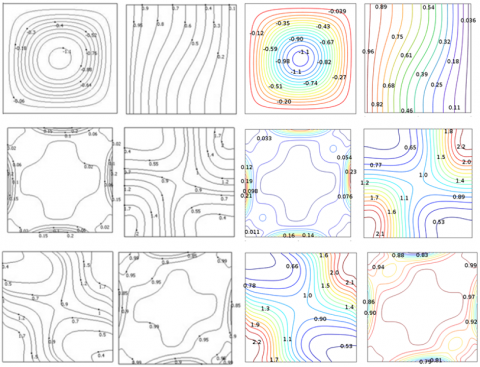Shavik et al., (2014)                           Present Work

Figure 2. Validation of the present work with Shavik etal

in terms of Stream function (first row), isotherm (second row), entropy generation due to fluid friction (third row), entropy generation due to heat transfer (fourth row), total entropy generation (fifth row) and Bejan number (sixth row) at Ra=104, φ=0.06

The finite element method  is almost exclusively used, in area mechanics of solid, the intensive research has encourage on its usage regarding the area of Computational Fluid Dynamics (CFD). the reseach recommend it as a powerful alternative scheme for ocean models beside that considering the variabels resolution, it allows the use of unstructured grids. In the subject of mathematics, espisially regarding the area of numerical solutions, Galerkin style were a class of techniques that converts a continuous operator issue (such as a differential equation) into a discrete issue. Galerkin's method supplies a powerful numerical scheme to differential equations and modal analysis. Figure 3 displays a two-dimensional computational zone for trapezoidal enclsoure with baffle in Cartesian coordinate system which is sub-split into a number of small elements of the triangle mesh.

## 3.png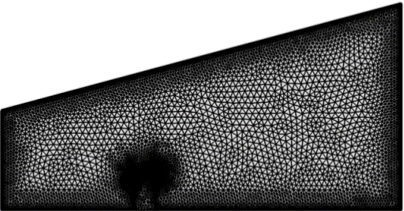Figure 3. Two dimensional numerical grid generation within the trapezidal enclsoure with attached buffle

## 4.pngFigure 4. convergence of the average Nusselt number along the hot left sidewall of the trapezoidal cavity

In order to get grid independent solution, a grid indepedent study is presented in Figure 4 for trapezidal enclsoure at Ra = 106, Hb=H*/3, φ = 0.06 for various number of elements (433, 673, 1045, 1854, 2832, 4580, 11553, 28964, 42700) of non-uniform grid are used to obtain the effect of numerical grid generation in the ecuracy of the average Nusselt number along the hot left sidewall of the trapezoidal cavity. As in Fig.4 when the number of elements are 11553 give accurate results and there are no need to increase the number of elments. So that we use this number of elment in the validation and the rest of the calculations.

4. Results and dissuasion

Figures 5-6 illustrate the streamlines (Ψ) and isotherms (θ), local entropy generation due to fluid friction, local entropy generation due to heat transfer $S_{\theta}$, total entropy generation $S_{\mathrm{L}}$ and Bejan number contours for different values of Rayleigh number and when [φ =0.06, Hb=H*/3, Lb=L/3]. It can be seen from Figuer 5 that that as the Rayleigh number increases from Ra = 104 into Ra = 106.

The maximum absolute value of the stream function increases from $\left[|\Psi|_{\max }=0.49\right]$ to $\left[|\Psi|_{\max }=11\right]$. The physical reason behind this is due to rapid movement of the fluid when the Rayleigh number value goes up. Since, the effect of buoyancy force and natural convection within the enclosure become very strong leading to rapidly increasing in the stream function values. With respect to the isotherms, it may be noted that at Ra=104 (low Rayleigh number) the conductive heat transfer is dominated and the shapes of isotherms are uniform because the weak effect of the convection. But, as the Rayleigh number increases to Ra = 106, an obvious change in isotherms shapes can be seen which can be taken as an indicator for increasing convection heat transfer rate. From the other hand the entropy generation maps illustrate that at low Rayleigh number [Ra=104] the entropy generation due to heat transfer is more dominated along the trapezoidal enclosure than the local entropy generation due to fluid friction $\left[S_{\theta}>S_{\Psi}\right]$ because the conduction heat transfer mode is more dominated mode rather than convection mode. For this reason the total entropy generation is similar to that of entropy generation due to heat transfer. Regarding the Bejan number maps it can be noticed that is very similar to the entropy generation due to fluid friction map as the value of Bejan number is less than 0.5.

At high value of Rayleigh number [Ra=106], it can be from the isotherm lines to become more curve lines which are an indicator on heat transfer enhancement by convection mode. It can be seen also, that due to increasing of natural convection and buoyancy force with an increasing Rayleigh number that map of total entropy generation indicates that $\left[S_{\Psi}>S_{\theta}\right]$. The Bejan number map is similar to that of entropy generation due to fluid friction map. With respect to the effect of baffle length on fluid flow and heat transfer, it can be seen from Figs.5-6 that it affects highly on stream function contours. For example, at low Rayleigh number [Ra=104] and at high Rayleigh number [Ra=106], the convection currents are divided into two inner circles when the baffle length increases from Hb=H*/3 into Hb=H*. Actually, these circles decreases the fluid flow strength for example when baffle length increases from Hb=H*/3 into Hb=H* at Ra = 106, the stream function decreases from $\left[|\Psi|_{\max }=11.17\right]$ into $\left[|\Psi|_{\max }=8.871\right]$. The baffle effects on isotherms is presented and it may be noted that at high Rayleigh number [Ra=106] that the convection current along the enclosure is change into curve lines but theses line is transformed into uniform line when it hits the baffle and flow cross it. This is because that baffle is a solid body and the heat transfer through it will be by conduction. This is why the isotherms line becomes uniform though the baffle.

## 5.png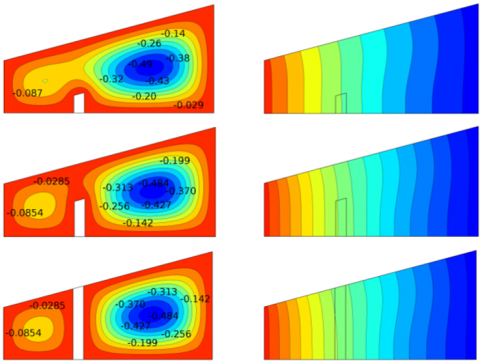Figure 5.a. Stream function (first row), isotherm (second row), at Ra=104, φ=0.06 for three cases

## 5b.pngFigure 5.b. entropy generation due to fluid friction (first row), entropy generation due to heat transfer (second row) Ra=104, φ=0.06 for three cases

## 5c.pngFigure 5.c. total entropy generation (first row), and Bejan (second row) Ra=104, φ=0.06 for three cases

## 6a.pngFigure 6.a. Stream function (first row), isotherm (second row), at Ra=106, φ=0.06 for three cases

## 6b.png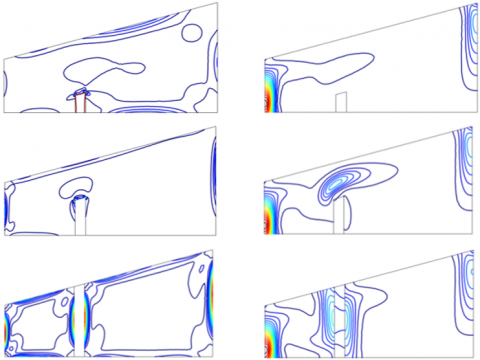Figure 6.b. Entropy generation due to fluid friction (first row), entropy generation due to heat transfer (second row) Ra=106, φ=0.06 for three cases

## 6c.pngFigure 6.c. total entropy generation (first row), and Bejan (second row) Ra=106, φ=0.06 for three cases

## 71.png## 72.png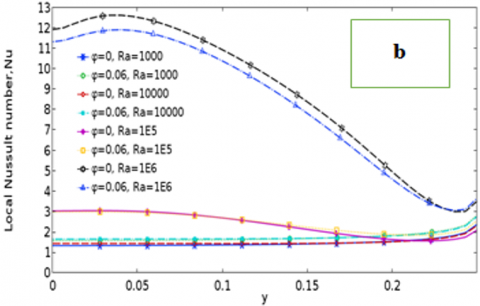## 73.pngFigure 7. The variation of local Nusselt numbers along hot surface at different Rayleigh numbers for three cases a) CASE-1 b) CASE-2 c) CAE-3

The baffle height on heat transfer rate is presented in Figure7 a, b and c in terms of local Nusselt number considering the three different cases for various Rayleigh number and solid particle volume fraction. From the first view it can be seen that the local Nusselt number profile decreases as the baffle height increases. Also, at low Rayleigh number there is a negligible change in the local Nusselt number profile except the sharp peak at the upper side of the hot wall/ at high value number, this sharp peak become more obvious at the lower and upper part of the hot wall. It is worth to mention that nanoparticle can enhance the heat transfer for CASE-1 and CASE-3 and it decrease it for CASE-2. It can be seen that as the baffle length increase, two inner circles will be formed within the enclosure and this will reduce the average Nusselt number leading to reduce the heat transfer rate as displayed in Figure 8. Also, it can be seen that the isotherms lines and at higher Rayleigh number [Ra = 106] become close to the baffle it will change their shapes from curve lines into uniform lines because the baffle is solid which makes the heat transferring this location is due to conduction mode. However, pass the baffle the isotherm lines will become curve lines again which indicate this fact. The baffle length of entropy generation is illustrated in Figure 9-11 and the main conclusion is that as the baffle length increase, all maps will decrease. However, there is negligible effect of baffle length on Bejan number as in Figure 12. From the above discussion, it can be seen that CASE-1 gives better heat transfer characteristics.

## 8.pngFigure 8. Variation of average Nusselt number with Rayleigh number under different cases of baffle length

## 9.png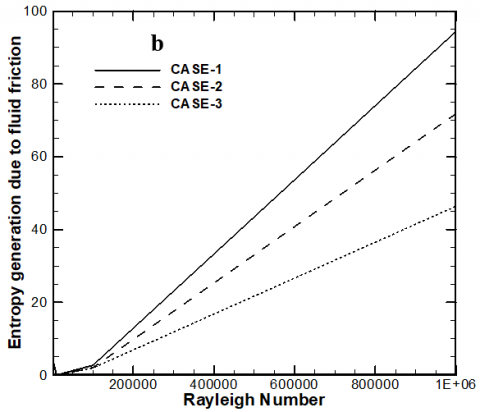Figure 9. Variation of Entropy generation due to fluid friction with Rayleigh number under different cases of baffle length

## 10.pngFigure 10. Variation of Entropy generation due to heat transfer with Rayleigh number under different cases of baffle length

## 113.png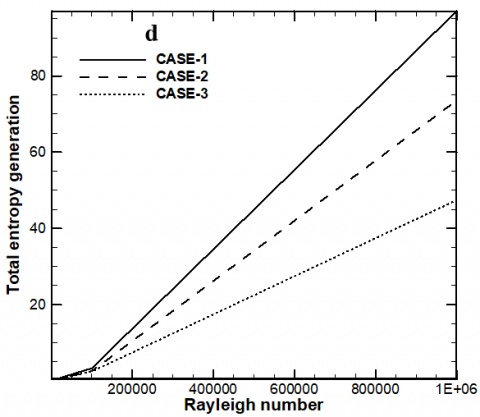Figure 11. Variation of Total Entropy generation with Rayleigh number under different cases of baffle length

## 12.pngFigure 12. Variation of Bejan number with Rayleigh number under different cases of baffle length

5. Conclusions

Numerical simulation using finite element approach had been used to study the two-dimesnional natural convection heat transfer inside trapezoidal enclosure filled with copper-water nanofluid. Three different cases of baffle height are studied in the present work and for various Rayleigh number and nanofluid concentration. The results indicate that increasing Rayleigh number will increase the fluid flow strength and heat transfer rate. Also, the baffle height has strong impact on streamlines, heat transfer and entropy generation contours and it is concluded that CASE-1 gives better heat transfer charcteristc more than other cases.

Nomenclature
 Cp Specific heat at constant pressure (KJ/kg.K) g Gravitational acceleration (m/s2) Nu Local Nusselt number k Thermal conductivity (W/m.K) $\overline{\mathrm{Nu}}$ Average Nusselt L Length of bottom wall of the cavity U Dimensionless velocity component in x-direction P Dimensionless pressure u Velocity component in x-direction (m/s) p Pressure (Pa) V Dimensionless velocity component in y-direction Pr Prandtl number (νf/αf) v Velocity component in y-direction (m/s) Ra Rayleigh number $\left(g \beta_{f} L^{3} \Delta T / v_{f} \alpha_{f}\right)$ X Dimensionless coordinate in horizontal direction T Temperature (K) x Cartesian coordinate in horizontal direction (m) Tc Temperature of the cold surface (K) Y Dimensionless coordinate in vertical direction Th Temperature of the hot surface (K) y Cartesian coordinate in vertical direction (m) Greek symbols α Inclination angle ν Kinematic viscosity (μ /ρ)(Pa. s) θ Dimensionless temperature   (T-Tc/ΔT) φ Nanoparticle volume fraction (%) Ψ Dimensional stream function (m2/s) ΔT Ref. temperature difference ψ Dimensionless stream function β Volumetric coefficient of thermal expansion (K-1) μ Dynamic viscosity (kg.s/m) ρ Density (kg/m3) Subscripts c Cold nf Nanofluid f Fluid (pure) s Source surface P Nanoparticle Abbreviations Min Minimum max Maximum
References

Abu-Nada E., Chamkha A. J. (2010). Effect of nanofluid variable properties on natural convection in enclosures filled with a CuO–EG–water nanofluid. International Journal of Thermal Sciences, Vol. 49, No. 12, pp. 2339-2352. https://doi.org/10.1016/j.ijthermalsci.2009.09.002

Abu-Nada E., Oztop H. F. (2010). Effects of inclination angle on natural convection in enclosures filled with Cu–water nanofluid. International Journal of Heat and Fluid Flow, Vol. 30, No. 4, pp. 669-678. https://doi.org/10.1016/j.ijheatfluidflow.2009.02.001

Altaç Z., Kurtul Ö. (2007). Natural convection in tilted rectangular enclosures with a vertically situated hot plate inside. Applied Thermal Engineering, Vol. 27, No. 11-12, pp. 1832-1840. https://doi.org/10.1016/j.applthermaleng.2007.01.006

Aminossadati S. M., Ghasemi B. (2009). Natural convection cooling of a localised heat source at the bottom of a nanofluid-filled enclosure. European Journal of Mechanics-B/Fluids, Vol. 28, No. 5, pp. 630-640. https://doi.org/10.1016/j.euromechflu.2009.05.006

Basak T., Anandalakshmi R., Kumar P., Roy S. (2012). Entropy generation vs energy flow due to natural convection in a trapezoidal cavity with isothermal and non-isothermal hot bottom wall. Energy, Vol. 37, No. 1, pp. 514-532.

Basak T., Chamkha A. J. (2012). Heatline analysis on natural convection for nanofluids confined within square cavities with various thermal boundary conditions. International Journal of Heat and Mass Transfer, Vol. 55, No. 21-22, pp. 5526-5543. https://doi.org/10.1016/j.ijheatmasstransfer.2012.05.025

Ben-Nakhi A., and Chamkha A. J. (2006). Effect of length and inclination of a thin fin on natural convection in a square enclosure. Numerical Heat Transfer, Vol. 40, No. 4, pp. 381-399. https://doi.org/10.1080/10407780600619907

Bilgen, E. (2005). Natural convection in cavities with a thin fin on the hot wall. International Journal of Heat and Mass Transfer, Vol. 48, No. 17, pp. 3493-3505. https://doi.org/10.1016/j.ijheatmasstransfer.2005.03.016

Bouhalleb M., Abbassi H. (2014). Natural convection of nanofluids in enclosures with low aspect ratios. International journal of hydrogen energy, Vol. 39, No. 27, pp. 15275-15286. https://doi.org/10.1016/j.ijhydene.2014.04.069

Chahrazed B., Samir R. (2012). Simulation of heat transfer in a square cavity with two fins attached to the hot wall. Energy Procedia, No. 18, pp. 1299-1306. https://doi.org/10.1016/j.egypro.2012.05.147

Cianfrini M., Corcione M., and Quintino A. (2015). Natural convection in square enclosures differentially heated at sides using alumina-water nanofluids with temperature-dependent physical properties. Thermal Science, Vol. 19, No. 2, pp. 591-608.

Davis de V. G. (1983) Natural convection of air in a square cavity: a bench mark numerical solution. International Journal for numerical methods in fluids, Vol. 3, No. 3, pp. 249-264. https://doi.org/10.1002/fld.1650030305

Fontana é., Silva A. d., Mariani V. C., Marrconded F. (2010) The influence of baffles on the natural convection in trapezoidal cavities. Numerical Heat Transfer, Part A: Applications, Vol. 58, No. 2, pp. 125-145. https://doi.org/10.1080/10407782.2010.496673

Ghasemi B., Aminossadati S. M. (2009). Natural convection heat transfer in an inclined enclosure filled with a water-CuO nanofluid. Numerical Heat Transfer, Part A: Applications, Vol. 55, No. 8, pp. 807-823. https://doi.org/10.1080/10407780902864623

Ho C.-J., Chen M. W., Li Z. W. (2008). Numerical simulation of natural convection of nanofluid in a square enclosure: effects due to uncertainties of viscosity and thermal conductivity. International Journal of Heat and Mass Transfer, Vol. 51, No. 17-18, pp. 4506-4516. https://doi.org/10.1016/j.ijheatmasstransfer.2007.12.019

Hussein A., Lioua K., Chand R., Sivasankaran S., Nikbakhti R., Li D., Naceur B., Habib B. (2016). Three-dimensional unsteady natural convection and entropy generation in an inclined cubical trapezoidal cavity with an isothermal bottom wall. Alexandria Engineering Journal, Vol. 55, No. 2, pp. 741-755. https://doi.org/10.1016/j.aej.2016.01.004

Iyican L., Witte L., Bayazitoglu Y. (1980). An experimental study of natural convection in trapezoidal enclosures. Journal of Heat Transfer, Vol. 102, No. 4, pp. 648-653.

Kahveci K. (2010). Buoyancy driven heat transfer of nanofluids in a tilted enclosure. Journal of Heat Transfer, Vol. 132, No. 6, pp. 062501.

Karki K. C. (1987). A Calculation Procedure For Viscous Flows At All Speeds In Complex Geometries. University of Minnesota .

Karyakin Y. E. (1989). Transient natural convection in prismatic enclosures of arbitrary cross-section. International journal of heat and mass transfer, Vol. 32, No. 6, pp. 1095-1103. https://doi.org/10.1016/0017-9310(89)90009-4

Kuyper R. A., Hoogendoorn C. J. (1995). Laminar natural convection flow in trapezoidal enclosures. Numerical Heat Transfer, Part A: Applications, Vol. 28, No. 1, pp. 55-67. https://doi.org/10.1080/10407789508913732

Lee T. S. (1991). Numerical experiments with fluid convection in tilted nonrectangular enclosures. Numerical Heat Transfer, Vol. 19, No. 4, pp. 487-499. https://doi.org/10.1080/10407789108944861

Mahmoodi M. (2011). Numerical simulation of free convection of nanofluid in a square cavity with an inside heater. International Journal of Thermal Sciences, Vol. 50, No. 11, pp. 2161-2175. https://doi.org/10.1016/j.ijthermalsci.2011.05.008

Mahmoodi M., Sebdani S. M. (2012). Natural convection in a square cavity containing a nanofluid and an adiabatic square block at the center. Superlattices and Microstructures, Vol. 52, No. 2, pp. 261-275. https://doi.org/10.1016/j.spmi.2012.05.007

Mahmoudi A. H., Pop I., Shahi M. (2013). MHD natural convection and entropy generation in a trapezoidal enclosure using Cu–water nanofluid. Computers & Fluids, No. 72, pp. 46-62. https://doi.org/10.1016/j.compfluid.2012.11.014

Mahmoudi A. H., Shahi M., Honarbakhsh-Raouf A., Ghasemian A. (2010). Numerical study of natural convection cooling of horizontal heat source mounted in a square cavity filled with nanofluid. International Communications in Heat and Mass Transfer, Vol. 37, No. 8, pp. 1135-1141. https://doi.org/10.1016/j.icheatmasstransfer.2010.06.005

Maxwell J. C., Thompson J. J. (1904). A treatise on electricity and magnetism. Vol. 2, Clarendon. https://doi.org/10.1017/CBO9780511709340

Moukalled F., Darwish M. (2003). Natural convection in a partitioned trapezoidal cavity heated from the side. Numerical Heat Transfer: Part A: Applications, Vol. 43, No. 5, pp. 543-563. https://doi.org/10.1080/10407780307313

Moukalled F., Darwish M. (2004). Natural convection in a trapezoidal enclosure heated from the side with a baffle mounted on its upper inclined surface. Heat transfer engineering, Vol. 25, No. 8, pp. 80-93. https://doi.org/10.1080/01457630490520356

Nag A., Sarkar A., and Sastri V. (1993). Natural convection in a differentially heated square cavity with a horizontal partition plate on the hot wall. Computer methods in applied mechanics and engineering, Vol. 110, No. 1-2, pp. 143-156. https://doi.org/10.1016/0045-7825(93)90025-S

Nasrin R., and Parvin S. (2012). Investigation of buoyancy-driven flow and heat transfer in a trapezoidal cavity filled with water–Cu nanofluid. International Communications in Heat and Mass Transfer, Vol. 39, No. 2, pp. 270-274. https://doi.org/10.1016/j.icheatmasstransfer.2011.11.004

Omid M., Ali K., Clement K. (2013). A review of entropy generation in nanofluid flow. International Journal of Heat and Mass Transfer, No. 65, pp. 514-532. https://doi.org/10.1016/j.ijheatmasstransfer.2013.06.010

Oztop H. F., and Abu-Nada E. (2008). Numerical study of natural convection in partially heated rectangular enclosures filled with nanofluids. International journal of heat and fluid flow, Vol. 29, No. 5, pp. 1326-1336. https://doi.org/10.1016/j.ijheatfluidflow.2008.04.009

Öztop H. F., Mobedi M., Abu-Nada E., Pop I. (2012) A heatline analysis of natural convection in a square inclined enclosure filled with a CuO nanofluid under non-uniform wall heating condition. International Journal of Heat and Mass Transfer, Vol. 55, No. 19-20, pp. 5076-5086. https://doi.org/10.1016/j.ijheatmasstransfer.2012.05.007

Oztop H., and Bilgen E. (2006). Natural convection in differentially heated and partially divided square cavities with internal heat generation. International journal of heat and fluid flow, Vol. 27, No. 3, pp. 466-475. https://doi.org/10.1016/j.ijheatfluidflow.2005.11.003

Perić M. (1993). Natural convection in trapezoidal cavities. Numerical Heat Transfer, Part A: Applications, Vol. 24, No. 2, pp. 213-219. https://doi.org/10.1080/10407789308902614

Ramakrishna D., Basak T., and Roy S. (2013). Analysis of heatlines and entropy generation during free convection within trapezoidal cavities. International Communications in Heat and Mass Transfer, No. 45, pp. 32-40. https://doi.org/10.1016/j.icheatmasstransfer.2013.04.004

Saleh H., Roslan R., and Hashim I. (2011). Natural convection heat transfer in a nanofluid-filled trapezoidal enclosure. International Journal of Heat and Mass Transfer, Vol. 54, No. 1-3, pp. 194-201. https://doi.org/10.1016/j.ijheatmasstransfer.2010.09.053

Santra A. K., Sen S., Chakraborty N. (2008). Study of heat transfer augmentation in a differentially heated square cavity using copper–water nanofluid. International Journal of Thermal Sciences, Vol. 47, No. 9, pp. 1113-1122. https://doi.org/10.1016/j.ijthermalsci.2007.10.005

Sathiyamoorthy M., Chamkha A. J. (2014). Analysis of natural convection in a square cavity with a thin partition for linearly heated side walls. International Journal of Numerical Methods for Heat & Fluid Flow, Vol. 24, No. 5, pp. 1057-1072. https://doi.org/10.1108/HFF-02-2012-0050

Selimefendigil F., and Öztop H. F. (2014). Estimation of the mixed convection heat transfer of a rotating cylinder in a vented cavity subjected to nanofluid by using generalized neural networks. Numerical Heat Transfer, Part A: Applications, Vol. 65, No. 2, pp. 165-185.

Selimefendigil F., and Öztop H. F. (2014). MHD mixed convection of nanofluid filled partially heated triangular enclosure with a rotating adiabatic cylinder. Journal of the Taiwan Institute of Chemical Engineers, Vol. 45, No. 5, pp. 2150-2162.

Selimefendigil F., and Öztop H. F. (2014). Numerical study of MHD mixed convection in a nanofluid filled lid driven square enclosure with a rotating cylinder. International journal of Heat and Mass Transfer, No. 78, pp. 741-754. https://doi.org/10.1016/j.ijheatmasstransfer.2014.07.031

Selimefendigil F., and Öztop H. F. (2016). Conjugate natural convection in a cavity with a conductive partition and filled with different nanofluids on different sides of the partition. Journal of Molecular Liquids, No. 216, pp. 67-77. https://doi.org/10.1016/j.molliq.2015.12.102

Shi X., Khodadadi J. (2003). Laminar natural convection heat transfer in a differentially heated square cavity due to a thin fin on the hot wall. Journal of Heat Transfer, Vol. 125, No. 4, pp. 624-634. https://doi.org/10.2514/6.2006-3094

Silva A. d., Fontana É., Mariani V. C., Marcondes F. (2012). Numerical investigation of several physical and geometric parameters in the natural convection into trapezoidal cavities. International Journal of Heat and Mass Transfer, Vol. 55, No. 23-24, pp. 6808-6818. https://doi.org/10.1016/j.ijheatmasstransfer.2012.06.088

Shavik S., Nasim H. M., Monjur M. A. (2014). Natural convection and entropy generation in a square inclined cavity with differentially heated vertical walls. Procedia Engineering, No. 90, pp. 557-562. https://doi.org/10.1016/j.proeng.2014.11.772

Ternik P., and Rudolf R. (2012). Heat transfer enhancement for natural convection flow of water-based nanofluids in a square enclosure. International Journal of Simulation Modelling, Vol. 11, No. 1, pp. 29-39